Home

# Magnetism – Proof of Concept, part 2

The preceding chapter showed that the Velocity Dependant Coulombs Law (VDCL) could be used to determine the exact result as Maxwell’s magnetic force equations. The example used however was simplified because it had the same current and cross-sectional area in each wire. Will the equations hold true in other situations? Here we’ll derive a general equation involving different currents and cross-sectional areas.

Consider the two wires in the below diagram: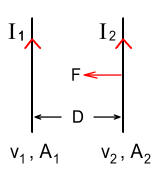Wire 1 (at left) has a current of I1 and a cross-sectional area A1. Wire 2 has a current of I2 and a cross-sectional area A2. What will be the force experienced by wire 2?

We are dealing with more variables now so the maths becomes more complex. Fortunately most of the work has already been done in the preceding chapter and it’s mostly a matter of substituting new values into the old equations.

Let the velocity of the electrons in wires 1 and 2 be v1 and v2 respectively. Since the electrons are moving at different velocities we now also need to determine the force between them. Previously this was unnecessary because they were motionless relative to each other.

So there are four forces to consider:
Fee = force from the electrons in wire 1 on the electrons in wire 2 (repels)
Fep = force from the electrons in wire 1 on the protons in wire 2 (attracts)
Fpe = force from the protons in wire 1 on the electrons in wire 2 (attracts)
Fpp = force from the protons in wire 1 on the protons in wire 2 (repels)

We’ll start with Fep.  As before, we determine the force on the protons in wire 2 from the electrons at +x and -x (in wire 1).  Taking the previous equations for Fp1 and Fp2; and replacing Fp1 with Fep1, Fp2 with Fep1, and v with v1 we get: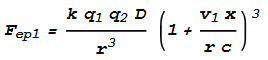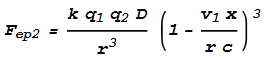Next we determine the force from the protons on the electrons, Fpe.  This will be same formula as Fep but with v1 replaced with v2: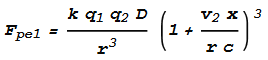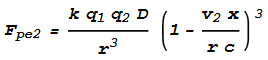Next to determine the forces between opposing electrons, Fee. Let the force from the electrons at +x and -x be Fee1 and Fee2 respectively. Taking the above equations for Fep, changing the force direction to negative, and replacing v1 with -(v1 - v2) (the negative difference in velocity) we get: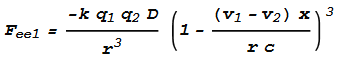Finally the force between protons, Fpp.  This can be determined from Fep1 + Fep2 by setting v1 to zero and changing force direction to negative. It is simply: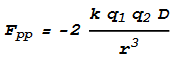The total force dF can be obtained by adding these seven forces together: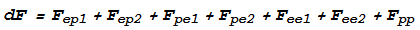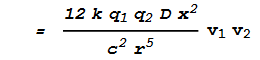Compare this with the corresponding formula in the previous chapter.  Notice that the only differences are that the result has increased by a factor of 2 (12 instead of 6) and v2 has been replaced with v1 v2.

The factor of 2 is due to looking at the force on the electrons in wire 2 from the protons wire 1 (Fpe), as well as the force on the protons in wire 2 from the electrons in wire 1 (Fep).  Whereas before we only looked at the force on the electrons in wire 2 from the protons in wire 1 (Fe).  In other words we have double counted.  To make this consistent with the previous chapter, we’ll reduce dF by a factor of 2: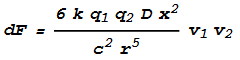Next we need to express the velocities in terms of current, charge density and area. This yields: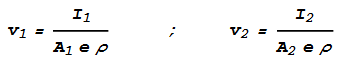To continue from the calculations in the previous chapter, q1 and q2 will become: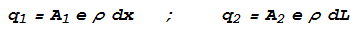Substituting this in gives: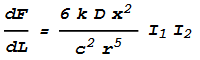Compare this with the corresponding formula in the previous chapter.  Notice the only difference is that I2 has been replaced with I1 I2.  Since this expression is a constant, we can skip the integration and substitute this expression into the final result.  This gives: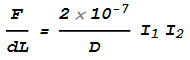Substituting the ‘permeability constant’ μ0 = 4*pi*10-7, the above can be written as: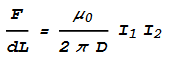Which is identical to the conventional magnetic force equation.

#### Conclusion

The Velocity Dependant Coulombs Law proposed here is able to match the conventional magnetic force equations in a general situation involving two wires carrying different currents, and this further indicates that the VDCL could be an accurate description of why magnetism occurs.

An added benefit is that we can use this method to determine the magnetic force in different situations.  For example, we can use it to determine magnetic force between two wires of finite length.  Because the conventional equation requires the ‘source wire’ to be infinitely long (while the ‘target wire’ can be any length), and this could never represent a real situation.

 Previous Contents Next chapter